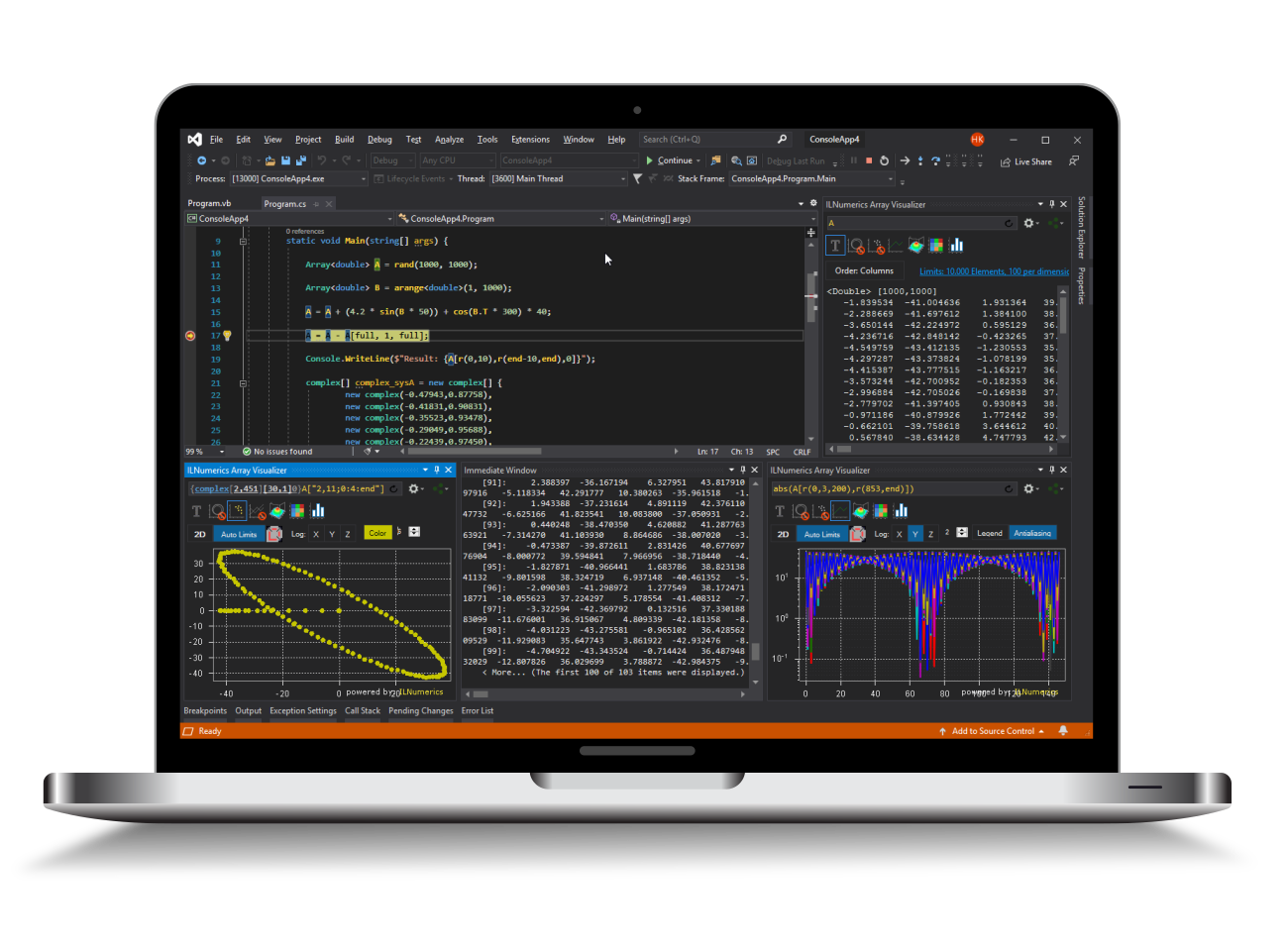Industrial Data Science
in C# and .NET:
Simple. Fast. Reliable.ILNumerics - Technical Computing

Modern High Performance Tools for Technical

Computing and Visualization in Industry and Science

tgt

# QR Decomposition in .NET (C#, Visual Basic)

The QR decomposition of a matrix real or complex matrix $A$ is a decompositioninto an orthonormal matrix $Q$ and an upper triangular matrix $R$ according to

$$A = Q \cdot R$$

Since $Q$ is orthonormal we can write

$$Q \cdot Q^* = I$$

where $I$ is the unit matrix and $Q^*$ represents the transpose and complex conjugate of $Q$.

## Using the QR Decomposition in .NET (C#, Visual Basic)

This piece of code represents the simplest call of qr. There are a number of overloads that allow for decomposition of complex matrices as well.

As an example we can also obtain the permutation matrix from the pivoting.

The boolean in the last call reduces the size of the problem if the matrix $A$ is rectangular.

The matrices fulfill the equation

$$A \cdot E = Q \cdot R$$

if the boolean is set to false. In this case $E$ is a matrix. If the boolean is set to true we have

$$A[":",E] = Q \cdot R$$

because $E$ is now a permutation vector.